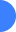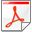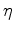Wave-equation migration velocity analysis for VTI media using optimized implicit finite differenceNext: Introduction Up: Reproducible Documents

# Wave-equation migration velocity analysis for VTI media using optimized implicit finite difference

Yunyue (Elita) Li

### Abstract:

Anisotropic wave-equation migration velocity analysis (WEMVA) requires fast and accurate wave modeling at all angles. I use an optimized implicit finite difference one-way propagation engine to improve both the efficiency and accuracy of this process. In this implicit finite difference scheme, anisotropic parametersandare mapped into two finite difference coefficients,and. When computing the perturbed wavefields from model perturbation, I apply a chain rule to link the wave equation with the actual anisotropic parameters via the finite difference coefficients. I test the implementation by impulse responses in both 2D and 3D. The sensitivity kernels for wave-equation reflection tomography confirm the theoretical understanding that waves have a higher sensitivity forat large angles and a higher sensitivity for vertical velocity at small angles.Wave-equation migration velocity analysis for VTI media using optimized implicit finite differenceNext: Introduction Up: Reproducible Documents

2012-05-10